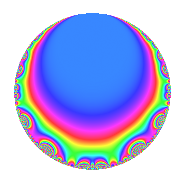# Properties

 Label 945.2.a.fLevel 945 Weight 2 Character orbit 945.a Self dual Yes Analytic conductor 7.546 Analytic rank 1 Dimension 2 CM No Inner twists 1

# Related objects

## Newspace parameters

 Level: $$N$$ = $$945 = 3^{3} \cdot 5 \cdot 7$$ Weight: $$k$$ = $$2$$ Character orbit: $$[\chi]$$ = 945.a (trivial)

## Newform invariants

 Self dual: Yes Analytic conductor: $$7.54586299101$$ Analytic rank: $$1$$ Dimension: $$2$$ Coefficient field: $$\Q(\sqrt{13})$$ Coefficient ring: $$\Z[a_1, a_2]$$ Coefficient ring index: $$1$$ Fricke sign: $$1$$ Sato-Tate group: $\mathrm{SU}(2)$

## $q$-expansion

Coefficients of the $$q$$-expansion are expressed in terms of $$\beta = \frac{1}{2}(1 + \sqrt{13})$$. We also show the integral $$q$$-expansion of the trace form.

 $$f(q)$$ $$=$$ $$q$$ $$- \beta q^{2}$$ $$+ ( 1 + \beta ) q^{4}$$ $$- q^{5}$$ $$+ q^{7}$$ $$-3 q^{8}$$ $$+O(q^{10})$$ $$q$$ $$- \beta q^{2}$$ $$+ ( 1 + \beta ) q^{4}$$ $$- q^{5}$$ $$+ q^{7}$$ $$-3 q^{8}$$ $$+ \beta q^{10}$$ $$-3 q^{11}$$ $$+ ( -1 + \beta ) q^{13}$$ $$- \beta q^{14}$$ $$+ ( -2 + \beta ) q^{16}$$ $$+ \beta q^{17}$$ $$+ ( -1 - \beta ) q^{19}$$ $$+ ( -1 - \beta ) q^{20}$$ $$+ 3 \beta q^{22}$$ $$+ ( -3 + \beta ) q^{23}$$ $$+ q^{25}$$ $$-3 q^{26}$$ $$+ ( 1 + \beta ) q^{28}$$ $$+ ( -3 - \beta ) q^{29}$$ $$+ ( -7 + 2 \beta ) q^{31}$$ $$+ ( 3 + \beta ) q^{32}$$ $$+ ( -3 - \beta ) q^{34}$$ $$- q^{35}$$ $$+ ( -1 + 2 \beta ) q^{37}$$ $$+ ( 3 + 2 \beta ) q^{38}$$ $$+ 3 q^{40}$$ $$+ 3 \beta q^{41}$$ $$+ ( 5 - 4 \beta ) q^{43}$$ $$+ ( -3 - 3 \beta ) q^{44}$$ $$+ ( -3 + 2 \beta ) q^{46}$$ $$+ ( -3 - 2 \beta ) q^{47}$$ $$+ q^{49}$$ $$- \beta q^{50}$$ $$+ ( 2 + \beta ) q^{52}$$ $$+ ( -6 + 5 \beta ) q^{53}$$ $$+ 3 q^{55}$$ $$-3 q^{56}$$ $$+ ( 3 + 4 \beta ) q^{58}$$ $$+ ( 3 - 4 \beta ) q^{59}$$ $$+ ( 5 - 7 \beta ) q^{61}$$ $$+ ( -6 + 5 \beta ) q^{62}$$ $$+ ( 1 - 6 \beta ) q^{64}$$ $$+ ( 1 - \beta ) q^{65}$$ $$+ ( 2 + 5 \beta ) q^{67}$$ $$+ ( 3 + 2 \beta ) q^{68}$$ $$+ \beta q^{70}$$ $$+ ( -9 + 3 \beta ) q^{71}$$ $$+ ( -1 - 4 \beta ) q^{73}$$ $$+ ( -6 - \beta ) q^{74}$$ $$+ ( -4 - 3 \beta ) q^{76}$$ $$-3 q^{77}$$ $$+ ( -10 - 3 \beta ) q^{79}$$ $$+ ( 2 - \beta ) q^{80}$$ $$+ ( -9 - 3 \beta ) q^{82}$$ $$+ ( 3 + 4 \beta ) q^{83}$$ $$- \beta q^{85}$$ $$+ ( 12 - \beta ) q^{86}$$ $$+ 9 q^{88}$$ $$+ ( 3 - 6 \beta ) q^{89}$$ $$+ ( -1 + \beta ) q^{91}$$ $$- \beta q^{92}$$ $$+ ( 6 + 5 \beta ) q^{94}$$ $$+ ( 1 + \beta ) q^{95}$$ $$+ ( 2 - 5 \beta ) q^{97}$$ $$- \beta q^{98}$$ $$+O(q^{100})$$ $$\operatorname{Tr}(f)(q)$$ $$=$$ $$2q$$ $$\mathstrut -\mathstrut q^{2}$$ $$\mathstrut +\mathstrut 3q^{4}$$ $$\mathstrut -\mathstrut 2q^{5}$$ $$\mathstrut +\mathstrut 2q^{7}$$ $$\mathstrut -\mathstrut 6q^{8}$$ $$\mathstrut +\mathstrut O(q^{10})$$ $$2q$$ $$\mathstrut -\mathstrut q^{2}$$ $$\mathstrut +\mathstrut 3q^{4}$$ $$\mathstrut -\mathstrut 2q^{5}$$ $$\mathstrut +\mathstrut 2q^{7}$$ $$\mathstrut -\mathstrut 6q^{8}$$ $$\mathstrut +\mathstrut q^{10}$$ $$\mathstrut -\mathstrut 6q^{11}$$ $$\mathstrut -\mathstrut q^{13}$$ $$\mathstrut -\mathstrut q^{14}$$ $$\mathstrut -\mathstrut 3q^{16}$$ $$\mathstrut +\mathstrut q^{17}$$ $$\mathstrut -\mathstrut 3q^{19}$$ $$\mathstrut -\mathstrut 3q^{20}$$ $$\mathstrut +\mathstrut 3q^{22}$$ $$\mathstrut -\mathstrut 5q^{23}$$ $$\mathstrut +\mathstrut 2q^{25}$$ $$\mathstrut -\mathstrut 6q^{26}$$ $$\mathstrut +\mathstrut 3q^{28}$$ $$\mathstrut -\mathstrut 7q^{29}$$ $$\mathstrut -\mathstrut 12q^{31}$$ $$\mathstrut +\mathstrut 7q^{32}$$ $$\mathstrut -\mathstrut 7q^{34}$$ $$\mathstrut -\mathstrut 2q^{35}$$ $$\mathstrut +\mathstrut 8q^{38}$$ $$\mathstrut +\mathstrut 6q^{40}$$ $$\mathstrut +\mathstrut 3q^{41}$$ $$\mathstrut +\mathstrut 6q^{43}$$ $$\mathstrut -\mathstrut 9q^{44}$$ $$\mathstrut -\mathstrut 4q^{46}$$ $$\mathstrut -\mathstrut 8q^{47}$$ $$\mathstrut +\mathstrut 2q^{49}$$ $$\mathstrut -\mathstrut q^{50}$$ $$\mathstrut +\mathstrut 5q^{52}$$ $$\mathstrut -\mathstrut 7q^{53}$$ $$\mathstrut +\mathstrut 6q^{55}$$ $$\mathstrut -\mathstrut 6q^{56}$$ $$\mathstrut +\mathstrut 10q^{58}$$ $$\mathstrut +\mathstrut 2q^{59}$$ $$\mathstrut +\mathstrut 3q^{61}$$ $$\mathstrut -\mathstrut 7q^{62}$$ $$\mathstrut -\mathstrut 4q^{64}$$ $$\mathstrut +\mathstrut q^{65}$$ $$\mathstrut +\mathstrut 9q^{67}$$ $$\mathstrut +\mathstrut 8q^{68}$$ $$\mathstrut +\mathstrut q^{70}$$ $$\mathstrut -\mathstrut 15q^{71}$$ $$\mathstrut -\mathstrut 6q^{73}$$ $$\mathstrut -\mathstrut 13q^{74}$$ $$\mathstrut -\mathstrut 11q^{76}$$ $$\mathstrut -\mathstrut 6q^{77}$$ $$\mathstrut -\mathstrut 23q^{79}$$ $$\mathstrut +\mathstrut 3q^{80}$$ $$\mathstrut -\mathstrut 21q^{82}$$ $$\mathstrut +\mathstrut 10q^{83}$$ $$\mathstrut -\mathstrut q^{85}$$ $$\mathstrut +\mathstrut 23q^{86}$$ $$\mathstrut +\mathstrut 18q^{88}$$ $$\mathstrut -\mathstrut q^{91}$$ $$\mathstrut -\mathstrut q^{92}$$ $$\mathstrut +\mathstrut 17q^{94}$$ $$\mathstrut +\mathstrut 3q^{95}$$ $$\mathstrut -\mathstrut q^{97}$$ $$\mathstrut -\mathstrut q^{98}$$ $$\mathstrut +\mathstrut O(q^{100})$$

## Embeddings

For each embedding $$\iota_m$$ of the coefficient field, the values $$\iota_m(a_n)$$ are shown below.

For more information on an embedded modular form you can click on its label.

Label $$\iota_m(\nu)$$ $$a_{2}$$ $$a_{3}$$ $$a_{4}$$ $$a_{5}$$ $$a_{6}$$ $$a_{7}$$ $$a_{8}$$ $$a_{9}$$ $$a_{10}$$
1.1
 2.30278 −1.30278
−2.30278 0 3.30278 −1.00000 0 1.00000 −3.00000 0 2.30278
1.2 1.30278 0 −0.302776 −1.00000 0 1.00000 −3.00000 0 −1.30278
 $$n$$: e.g. 2-40 or 990-1000 Significant digits: Format: Complex embeddings Normalized embeddings Satake parameters Satake angles

## Inner twists

This newform does not admit any (nontrivial) inner twists.

## Atkin-Lehner signs

$$p$$ Sign
$$3$$ $$-1$$
$$5$$ $$1$$
$$7$$ $$-1$$

## Hecke kernels

This newform can be constructed as the intersection of the kernels of the following linear operators acting on $$S_{2}^{\mathrm{new}}(\Gamma_0(945))$$:

 $$T_{2}^{2}$$ $$\mathstrut +\mathstrut T_{2}$$ $$\mathstrut -\mathstrut 3$$ $$T_{11}$$ $$\mathstrut +\mathstrut 3$$